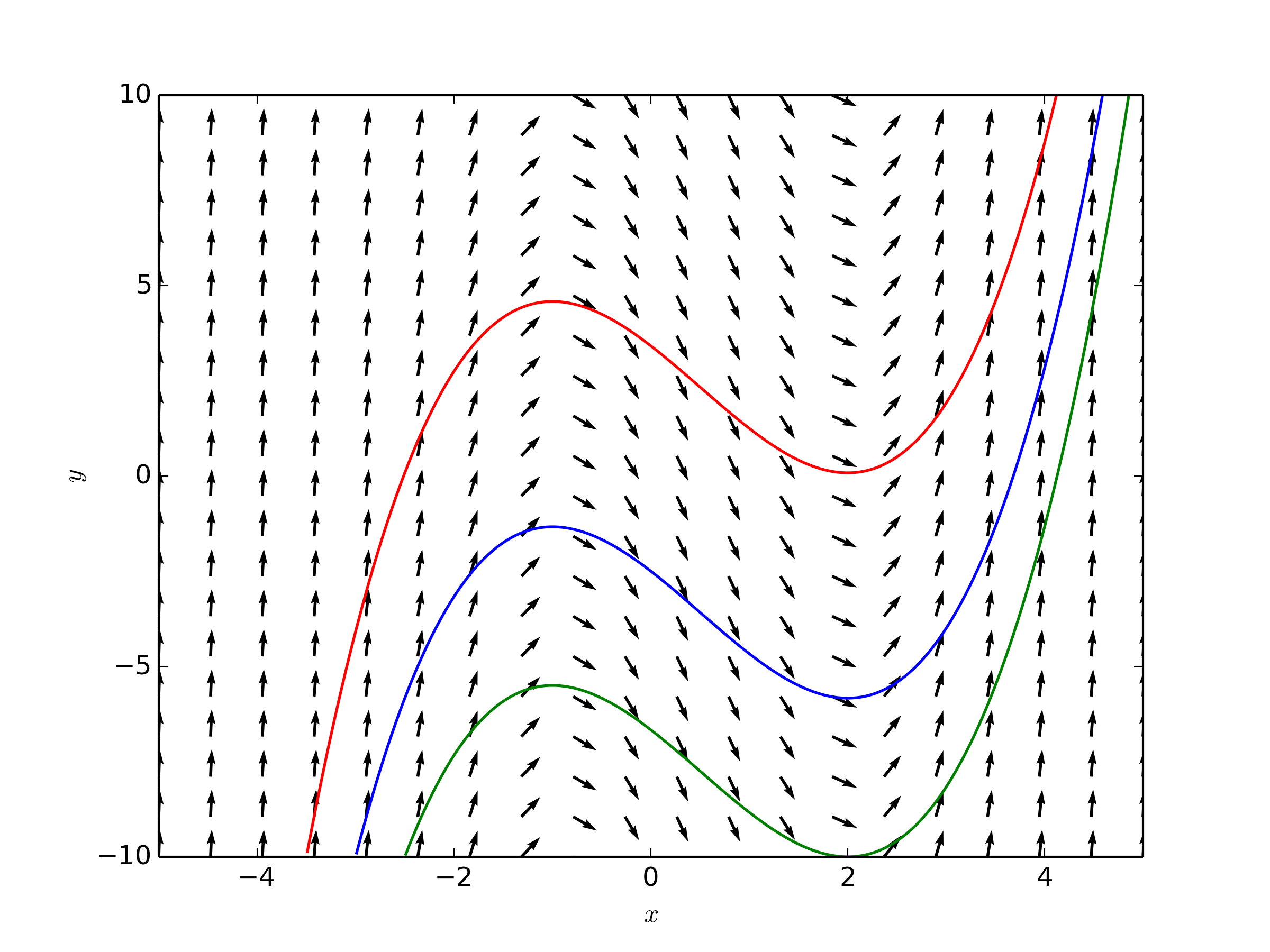## Direction Fields

A direction field shows the slope of the DE $$y^{'} = f(x,y)$$ at each point $$(x,y)$$. The figure below shows an example direction field. At each point $$(x,y)$$, a lineal element is shown depicting the slope. The three solutions below follow the direction of the fields for different particular solutions.Sketch the direction field for the equation $$\frac{dy}{dx}=-2xy$$. Sketch the solutions subject to $$y(0)=3$$.